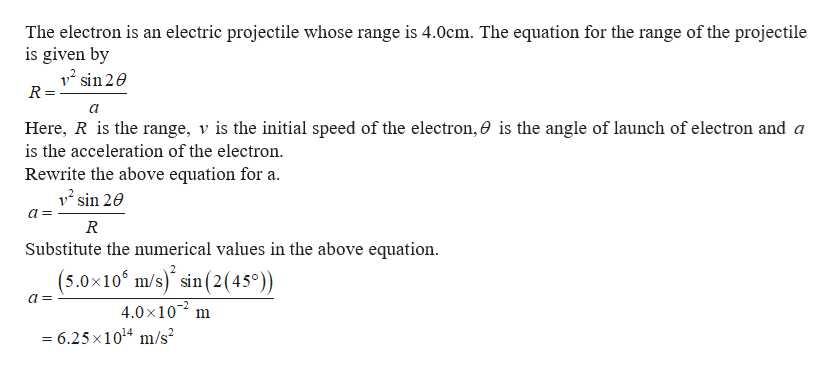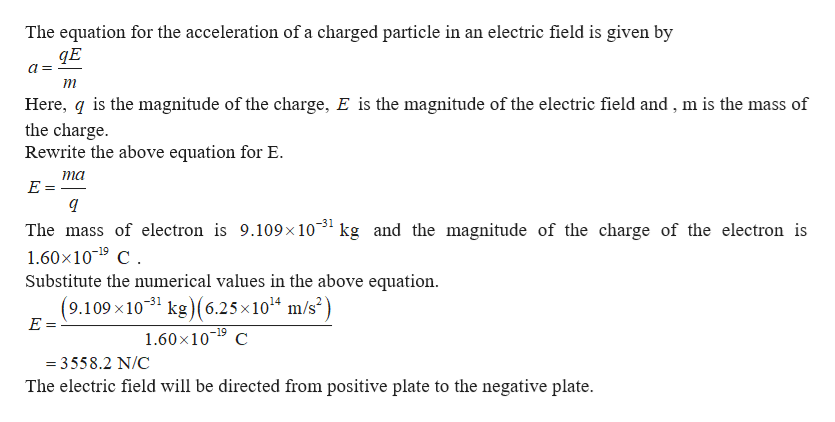450+++++++++ + +4.0 cm

Question

An electron is launched at a 45∘ angle and a speed of 5.0×106m/s from the positive plate of the parallel-plate capacitor shown in the figure (Figure 1). The electron lands 4.0 cm away.

What is the electric field strength inside the capacitor?

What is the smallest possible spacing between the plates?

Express your answer with the appropriate units.

Step 1help_outlineImage TranscriptioncloseThe electron is an electric projectile whose range is 4.0cm. The equation for the range of the projectile is given by 12 sin 2e R = Here, R is the range, v is the initial speed of the electron, 0 is the angle of launch of electron and a is the acceleration of the electron Rewrite the above equation for a 12 sin 20 R Substitute the numerical values in the above equation (5.0x10 m/s) sin(2 45) 4.0x10 = 6.25 x1014 m/s fullscreen
Step 2help_outlineImage TranscriptioncloseThe equation for the acceleration of a charged particle in an electric field is given by a Here, q is the magnitude of the charge, E is the magnitude of the electric field and, m is the mass of the charge Rewrite the above equation for E. ma E = The mass of electron is 9.109x1031 kg and the magnitude of the charge of the electron is 1.60x109 C Substitute the numerical values in the above equation (9.109 x1031 kg(6.25x1014 m/s2) E = -19 1.60x109 C =3558.2 N/C The electric field will be directed from positive plate to the negative plate fullscreen

Want to see the full answer?

See Solution

Want to see this answer and more?

Our solutions are written by experts, many with advanced degrees, and available 24/7

See Solution
Tagged in

Electric Charges and Fields